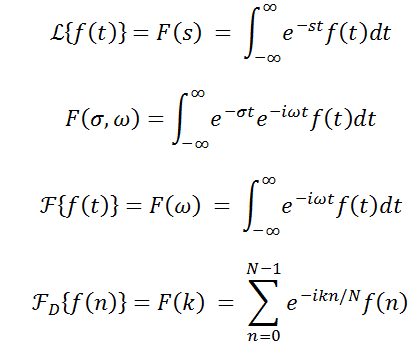# Inverse Discrete Laplace Transform

• swraman

#### swraman

Hi,

I have an idea which when tested looks like its clearly flawed. I am hoping someone can tell me where my procedure is flawed, or point me to some other theory that has already done something similar.The first two are the laplace transform.
The third line is the Fourier Transform.
The last line is teh Discrete Fourier transform.

Im looking at lines 2 and 4 (along with how 3 - the Fourier transform is related to 4). Is there anything stopping me from modifying the Discrete Fourier transform by adding in an extra variable sigma to the loop - an exp(-sigma*n/N) term - and iterating over a range of sigmas. That is, each Sigma would result in one "DFT".

If I do this process with an input time function h(t) equal to the impulse response of a system - that is compute the "DFT" over a range of sigma's. Then, if I place each of these "DFT"s together into a surface plot, should it not look like the transfer function plot of the system (represented by impulse response h(t) )?

I would expect to see the magnitude of the "DFT"s to spike to infinity at the value of sigma and omega representing the poles of the system whose impulse response is h(t). I used a simple 2-DOF system to generate an impulse response, then ran this process across a range of sigmas (my natural frequency was well within my sampling parameters' capability). But as I said, the result doesn't look like I expected..

Is there something incorrect with my thinking here?

Thanks

Last edited: